Previous: 4.3 Implementation in COMSOL    Top: Home    Next: 5.2 Interface Vacancy Pile-Up

### 5 Results and Discussion

The reliability of interconnects in ICs is a key issue for the overall quality of electronic systems. In this section the assessment of a structure called open TSV is presented.
Open TSVs are 3D structures made out of different materials, which exhibit corners and interfaces. These regions suffer from current crowding which breaks the homogeneity of the current density. Thereby, the available cross section is not optimally used. Furthermore, the interfaces between the different conducting layers show a behavior which promotes the EM induced effects. Therefore, current crowding is of major interest and analyzed in detail. This is followed by a full EM study of this structure regarding the most prominent locations, where EM leads to a failure. This failure is examined for two different modes. The first mode is the so called early mode, where a crack leads to an open circuit and an immediate failure. In the second so called late failure mode not a crack but a void is formed. This does not lead to an instant failure, but initializes a phase where the void grows and evolves. This evolution leads to a failure as soon as the void has grown big enough to block a significant part of the current conducting cross section, leading to an open circuit or a resistance increase over a critical value. These results are fitted to the generalized Black equation which is a widely used compact model for EM .

#### 5.1  Current Crowding

Current crowding is the effect of peaks in the current density due to locations of nonhomogeneous material parameters (e.g. lower resistance regions) or areas of elevated electrical field strength. These effects lead in metals to areas with raised Joule heating, which influence the material parameters and their behavior (e.g. Kirkendall effect ).
Therefore, these areas are particularly studied for open TSV structures with respect to the geometry and the resistance of the conducting materials.

Corners are typical regions where current crowding occurs. Sabelka  gave an analytic solution for a corner with an arbitrary angleshown in Figure 5.1.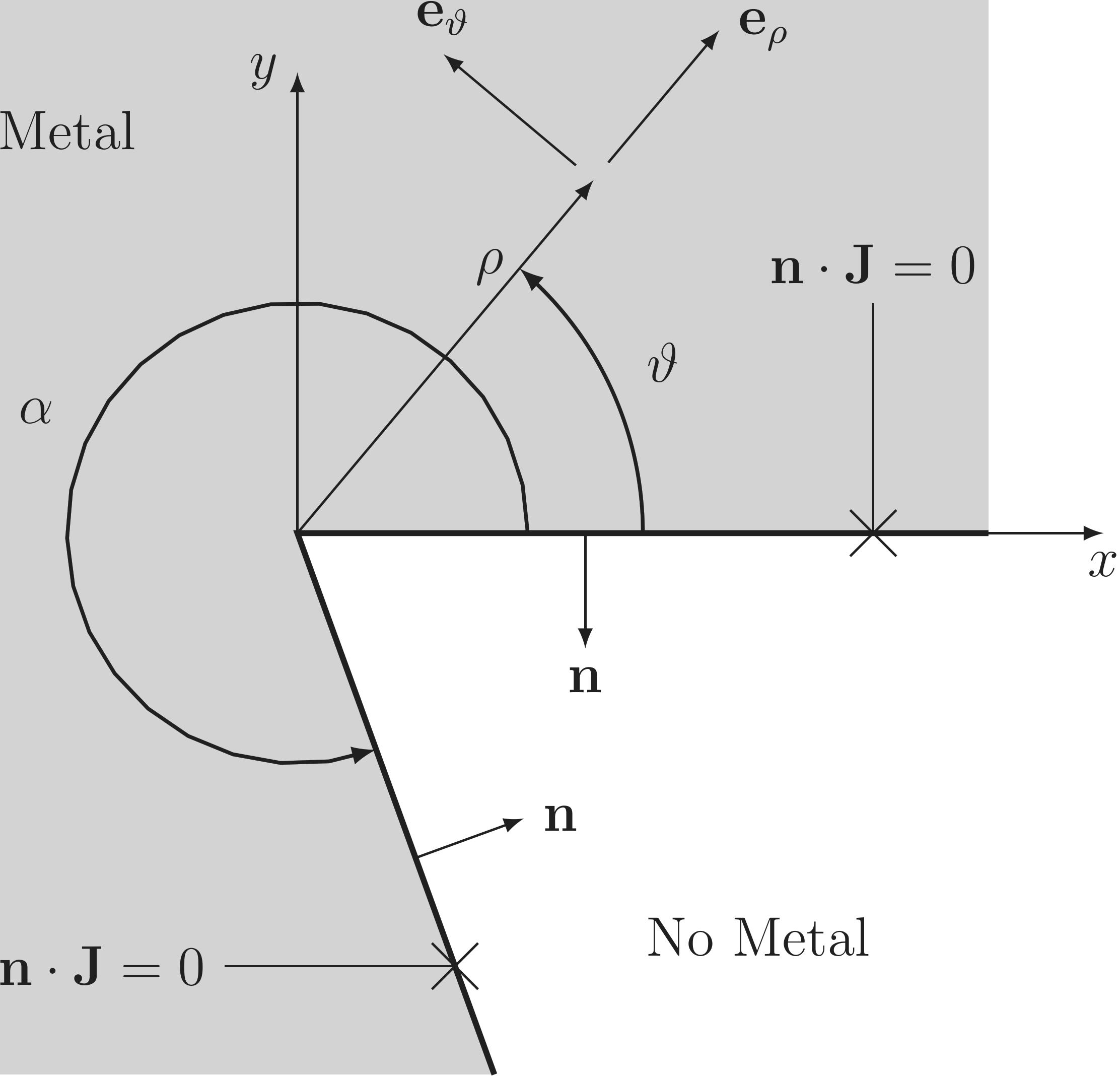Figure 5.1.:  Schematics of the current crowding calculation at a corner.

For this geometry the Poisson equation can be written in polar coordinates for two dimensions .This equation can be transformed intowhich allows the use of the ansatzleading to two separate equations depending only on one of the independent variablesandThe solution for the first differential equation is given bywhere the application of the boundary conditions, no normal flux at the metal edges (cf. Figure 5.1),for the anglesandonly allows solutions of the form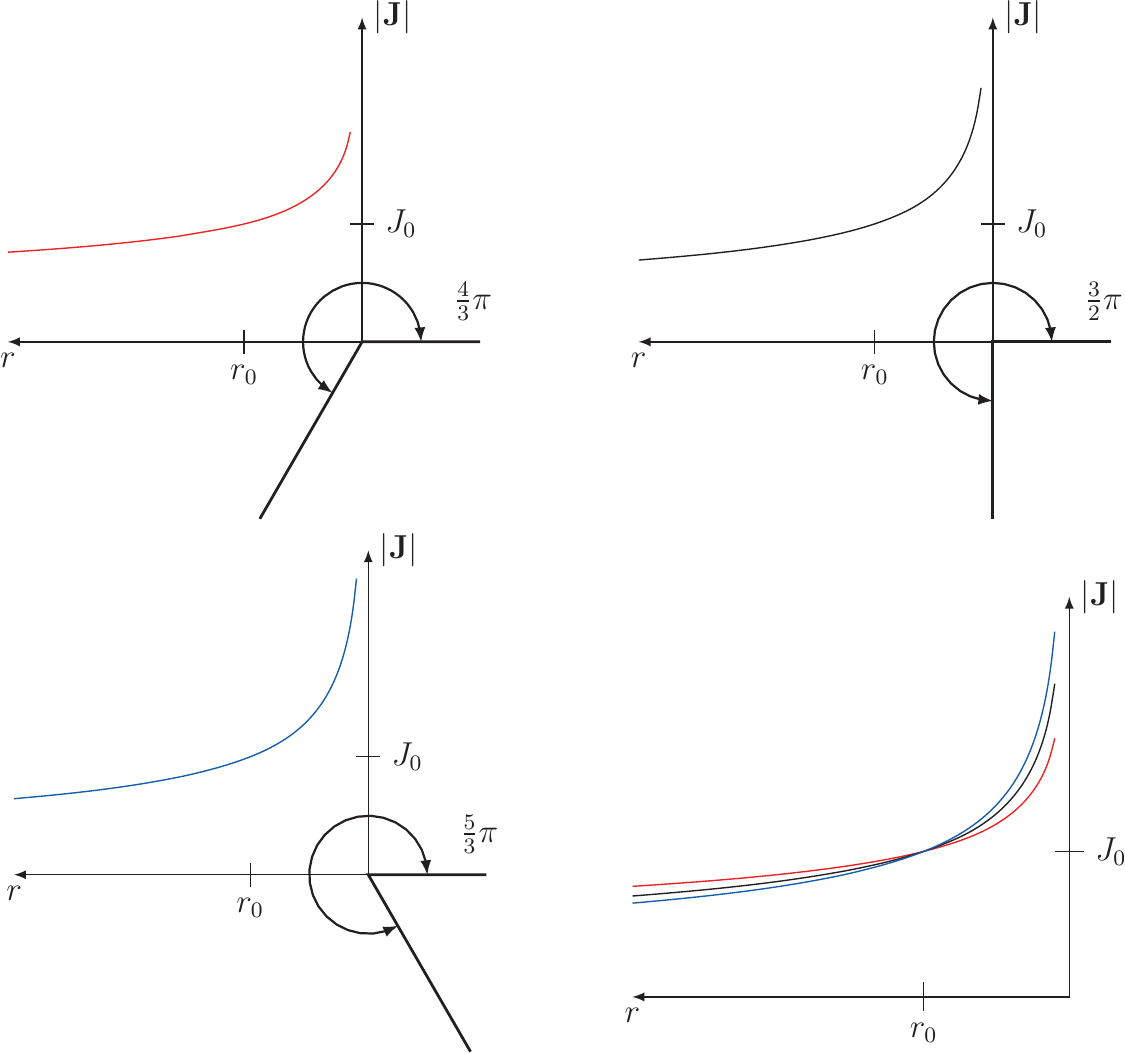Figure 5.2.:  The current density at corners for different angles versus the radial distance. The current densities are normalized to at the radius . The lower the angle of the corner, the higher the current density rises in the vicinity of the corner. In the last plot the three curves are put together to facilitate comparison.

For the second equation withthe solution has the formwhere only the first term has a physical meaning as the second has a singularity at the origin. Putting (5.8) and (5.9) together results inwithand the gradient for positive non-zerois given byThe gained solutions can show a singularity at the origin, where the exponent ofgets negative. This is true for those sections wheremeets the conditionwhich is only possible, whenis betweenand two timesandequals one. The norm of the current density foris given byFigure 5.2 shows the radial dependence of the current density for different angles. As the exponent of the equation points out, corners with higher angles show higher current densities in the vicinity of the corner, which is called current crowding.

In conventional vias and TSVs the whole vertically connecting structure is filled by the conducting metal. At present there are two kinds of material systems in use. The first one is the copper system, where the vertical and the horizontal connections are made out of copper, and the second is the aluminium tungsten system, where the horizontal or planar connections are made out of aluminium and the vertically connecting structures are made out of tungsten. For the later configuration, Figure 5.3 shows the current density at the interface of the planar interconnect and the via for different ratios of the via height and the via width. For higher ratios the current density at the interface between the horizontal interconnect and the vertical structure has a higher variation than for lower ratios. The highest current densities are found at the edges of the structures, where the right corner values are significantly higher compared to the left ones. In Figure 5.3a the current at the right corner is 3.5 times larger compared to the left corner.

The open TSV  is constructed as a hollow cylinder consisting of a tungsten coating, where at the top an aluminium cylinder is placed, overlapping the tungsten from the inside. Figure 5.4 depicts the structure, where the aluminium is shown in yellow, the tungsten in green, and the surrounding isolation layers and the silicon substrate in red.Figure 5.3.:  The current density is presented by the red arrows at the interface between the planar connecting structure and the via, for different ratios of the via width and the via height. The lines represent the electric flow lines.Figure 5.4.:  Profile view of the TSV structure: Aluminium in yellow, tungsten in green, and substrate and seal layer in red. The tungsten cylinder is shortened to 10% of the real length.

Cutting out the segment of interest results in the piece shown in Figure 5.5, where the curvature of the cylinder is neglected. This is a permissible assumption as the ratio of the layer thicknesses and the radius of the whole open TSV is in the order of 1 to 100.
Therefore, this structure was chosen for the simulations of the current crowding behavior at the aluminium/tungsten interface. Furthermore, the corner at the top of the TSV was not taken into account, as the results are only slightly changed by it.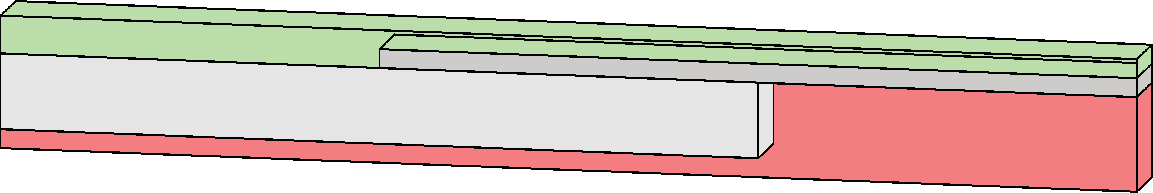Figure 5.5.:  The structure of a segment of the overlapping area of the tungsten cylinder and the aluminium cylinder. Green represents titanium nitride, red silicon oxide, dark gray tungsten, and light gray aluminium.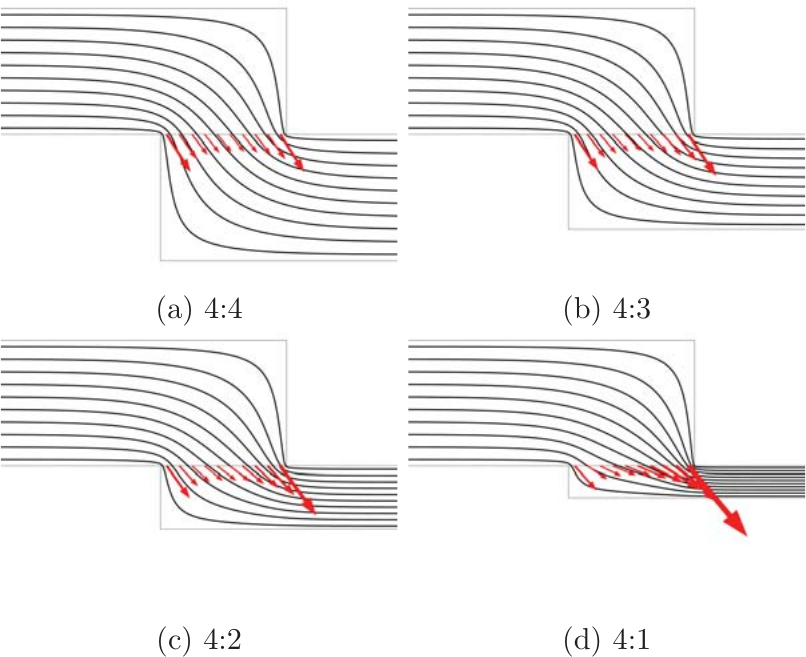Figure 5.6.:  The current density is shown by the red arrows at the interface between the aluminium layer on the top and the tungsten layer at the bottom for different ratios of the thicknesses of the two layers. The lines represent the electric flow lines.

The open TSV structure has a tungsten layer with a different thickness than the aluminium layer. Figure 5.6 shows the current densities, where the thickness of the aluminium layer on the top is kept constant and the thickness of the tungsten layer at the bottom is varied. There the assumption of equal conductivities is chosen to particularly see the impact of different thicknesses. In Figure 5.6 it can be seen that the current crowding takes place on both edges in the same range for a ratio of 1, as the red current density arrows are equal at both edges (cf. Figure 5.6). The smaller the thickness of the tungsten, the more current is transferred at the right corner, whereas the current crowding at the left corner gets smaller. In the studied TSV structure the ratio is in the range of 4, which is the reason that the higher current crowding takes place at the right corner.Figure 5.7.:  The current density is shown by the red arrows at the interface between the planar connecting structure and the via for different ratios of the resistances of the upper and the lower layer. The lines represent the electric flow lines.

In the simulations for different thicknesses the assumption of equal conductivity was chosen. This is in the case of tungsten and aluminium not true as tungsten has approximately half the conductivity of aluminium. In Figure 5.7 the conductivity of the aluminium is varied in multiples of the conductivity of tungsten with both layers having the same thickness to examine only the influence of resistance differences. The black lines are the current streamlines and the red arrows show the current density close to the interface. The upper ones show the current density in the aluminium and the lower ones show the same in the tungsten. The higher the ratio, the more deviation is present in the current densities between the materials. Furthermore, the current crowding is shifted to the right corner, the higher the ratio gets. Therefore, the current transfer between the two metals mainly occurs at the right side of the interface due to its special geometry on one hand and the difference in conductivities on the other hand, which leads to the high current crowding depicted in Figure 5.8.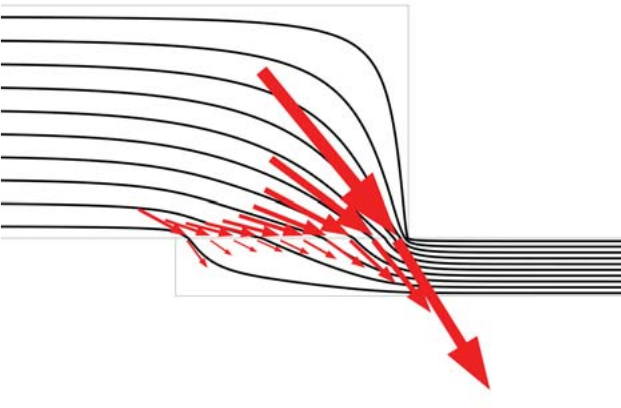Figure 5.8.:  The current density is shown by the red arrows at the interface between the planar connecting structure and the via. The lines represent the electric flow lines and show that the transfer of current between the two metals is mainly occurring closed to the right interface end.

The current densities at the ends of the interface show a saturation in relation to the interface length. For short interfaces the transfer of current between the two metals takes place over the whole interface length, whereas for an interface length over a characteristic lengththe middle part of the interface carries only current densities parallel to the interface and therefore, does not contribute to the current transfer between the metals.
The characteristic lengthis in the range of six times the layer thickness. In Figure 5.9 the current density component normal to the interface is depicted, showing this behavior in the middle of the interface.Figure 5.9.:  Component of the current density vector normal to the interface.
Previous: 4.3 Implementation in COMSOL    Top: Home    Next: 5.2 Interface Vacancy Pile-Up